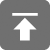# 工学1号馆

home

## tp5.1中Db类和模型的正确使用姿势

By Wu Yudong on April 25, 2019

## Db的正确姿势

// 查询单个数据
Db::name('user')->where('id', 1)->find();
// 查询多个数据
Db::name('user')->where('id', '>', 1)->select();
// 写入新的数据
Db::name('user')->insert(['name' => '张三']);
// 更新数据
Db::name('user')->where('id', 1)->update(['name' => '李四']);
// 删除数据
Db::name('user')->delete(1);

// 错误的用法

$user = Db::name('user'); // 查询单个数据$user->where('id', 1)->find();
// 查询多个数据
$user->where('id', '>', 1)->select(); // 写入新的数据$user->insert(['name' => '张三']);
// 更新数据
$user->update(['name' => '李四']); // 删除数据$user->delete(1);

// 仍然是错误的用法

// 查询单个数据
db('user')->where('id', 1)->find();
// 查询多个数据
db('user')->where('id', '>', 1)->select();
// 写入新的数据
db('user')->insert(['name' => '张三']);
// 更新数据
db('user')->update(['name' => '李四']);
// 删除数据
db('user')->delete(1);

$user = Db::name('user'); // 查询分数大于80分的用户总数$count = $user->where('score', '>', 80)->count(); // 查询分数大于80分的用户数据$user->select();

## 模型的正确姿势

// 写入新的数据
$user = User::create(['name' => '张三']); // 更新数据$user->update(['name' => '李四']);

// 查询单个数据
$user = User::get(1); // 删除当前模型数据$user->delete();

$user = new User; // 写入新的数据$user->name = '张三';
$user->save();$user = new User;
$user->find(1); echo$user->name;

// 写入新的数据
User::create(['name' => '张三']);
$user = User::get(1); echo$user->name;

### Comments

No comments yet.